Math resources Measurement and data

# Measurement and data

Here you will find all the measurement and data resources you need. Like all our math topic guides, we’ve included step-by-step guides, detailed examples and practice questions!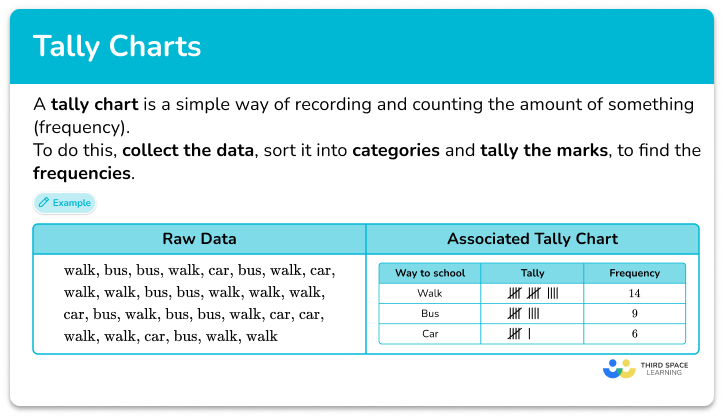Tally chart

Tally chart step-by-step topic guide, detailed examples, practice questions, teaching tips and FAQs.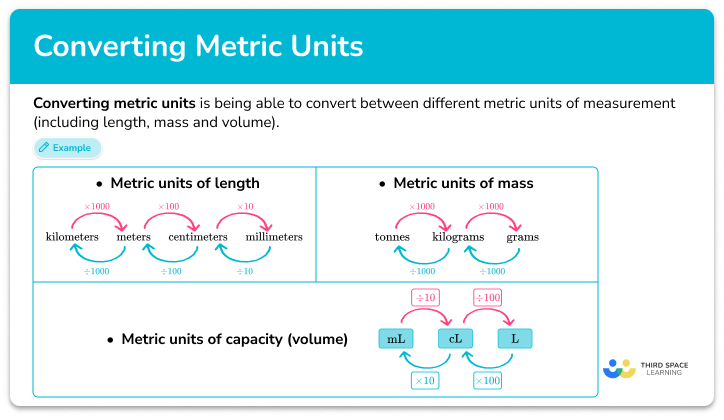Converting metric units

Converting metric units step-by-step topic guide, detailed examples, practice questions, teaching tips and FAQs.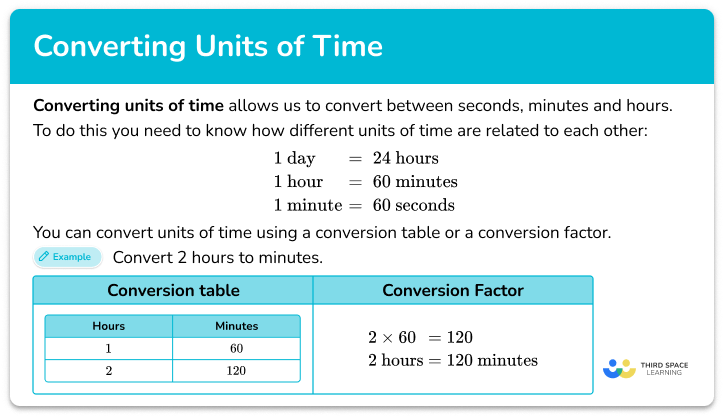Converting units of time

Converting units of time step-by-step topic guide, detailed examples, practice questions, teaching tips and FAQs.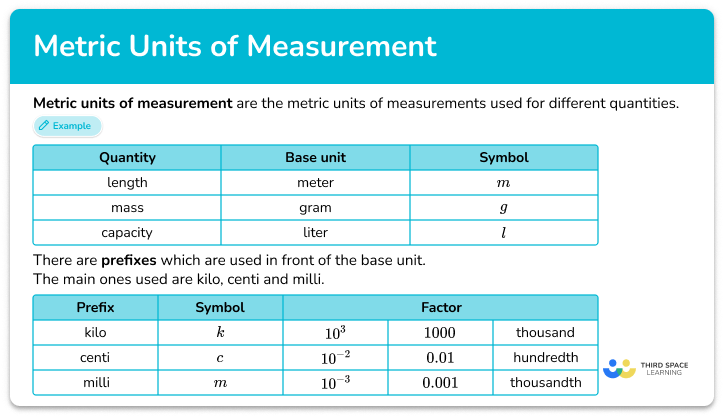Metric units of measurement

Metric units of measurement step-by-step topic guide, detailed examples, practice questions, teaching tips and FAQs.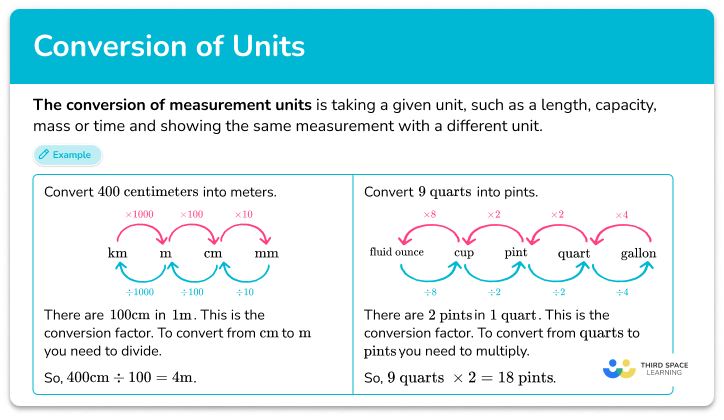Conversion of measurement units

Conversion of measurement units step-by-step topic guide, detailed examples, practice questions, teaching tips and FAQs.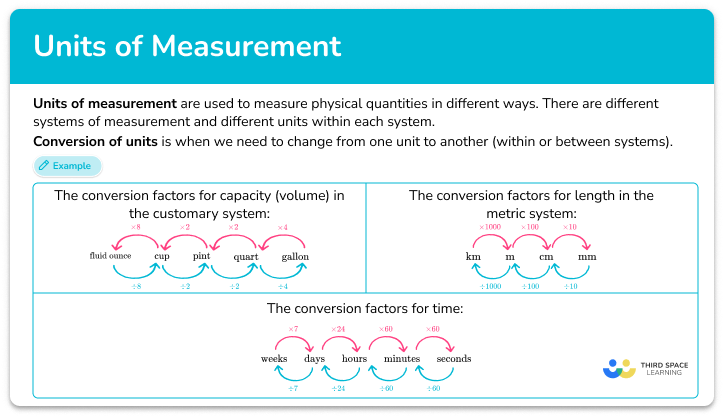Units of measurement

Units of measurement step-by-step topic guide, detailed examples, practice questions, teaching tips and FAQs.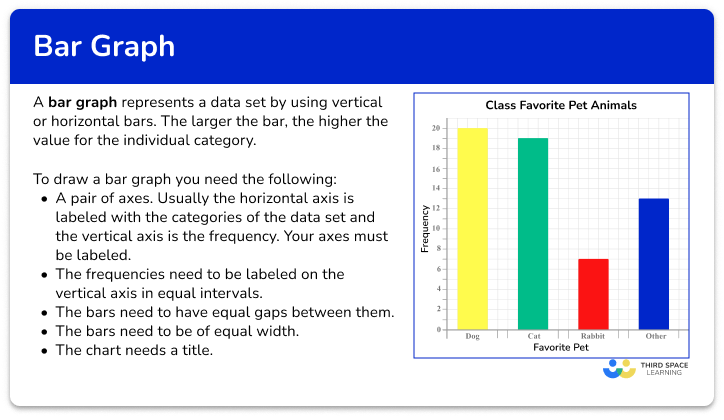Bar graph

Bar graph step-by-step topic guide, detailed examples, practice questions, teaching tips and FAQs.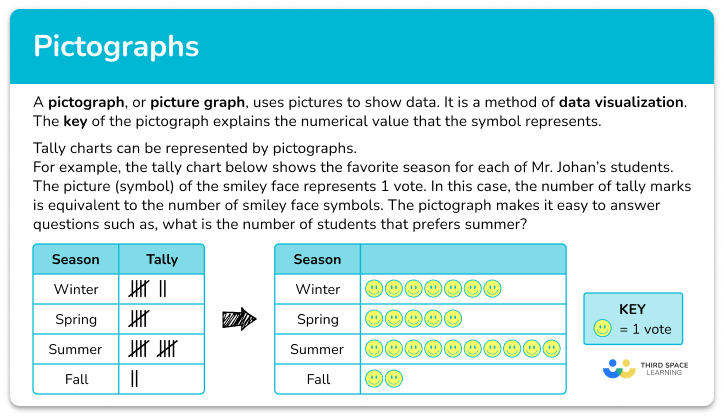Pictograph

Pictograph step-by-step topic guide, detailed examples, practice questions, teaching tips and FAQs.